# rand()The rand() function outputs a random number. there are two optional parameters that you can add to set mi and max for the number.<?phpecho rand();echo '<br>';echo rand(10,20);?>Result:```str_replace()
This function is useful when you want to do a simple match and replace.
This function accepts four parameters,
three required. find, replace, string, count(optional).
<?php
\$string = 'aaa-bbb-ccc';
echo str_replace('-', '*', \$string);
?>
Result:date()
The date() function can have two parameters,
format(required) and timestamp(optional).
<?php
echo date("d-m-Y h:i:s");
?>
Result: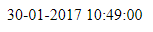strlen()
The strlen function will return the length of a given string.
The spaces and characters are added to the count.<?php
\$stringOne = "Hello PHP";
echo 'stringOne Length = ' . strlen(\$stringOne) . '<br><br>';
\$stringTwo = " Welcome code";
echo 'stringTwo Length = ' . strlen(\$stringTwo);
?>
Result:count()
This function count the number of element in a array.
<?php
\$Alphabets = array("A", "B", "C", "D", "E");
echo 'Count = '.count(\$Alphabets);
?>
Result: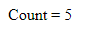array_combine()
This function creates an array by using
one array for keys and another for its values.
<?php
\$fruit_color = array("green", "red", "yellow");
\$fruits_array= array_combine(\$fruit_color, \$fruits);
print_r(\$fruits_array);
?>
Result:array_unique()
You can use this function to removes duplicate values from an array.
<?php
\$input = array("a" => "green", "red", "b" => "green", "blue", "red");
\$result = array_unique(\$input);
print_r(\$result);
?>
Result: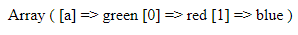print_r()
This function is used to print array variable in a way that’s readable by humans.
<?php
\$fruits = array ("a" => "Banana", "b" => "Apple");
print_r (\$fruits);
?>
Result:strrev()
This function reverses the given string.
<?php
echo strrev("Hello PHP");
?>
Result: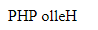trim()
This function removes characters, space from the string form both the ends.
<?php
\$str = "Hello PHPJavaScript";
echo \$str . "<br>";
echo trim(\$str,"Ht");
?>
Result: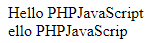ucfirst()
This function Converts the first character to uppercase.
<?php
echo ucfirst("hello php!");
?>
Result:For all PHP built in functions, go to  PHP.NET
Please do share and fallow our blog for more intresting content.

```